# 6.1 Exponential Functions

24 de Jan de 2023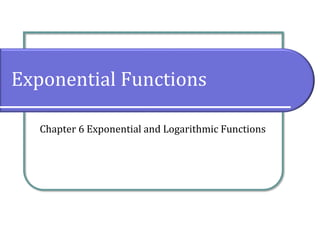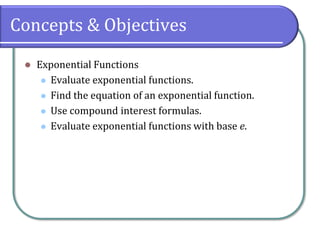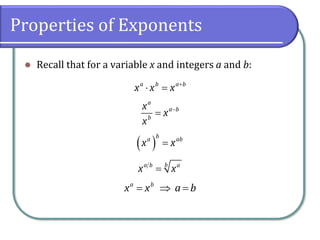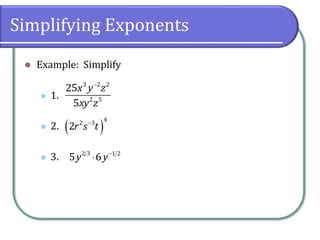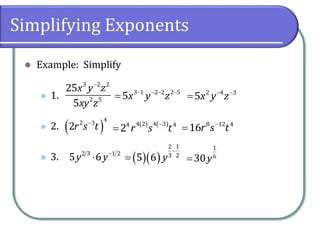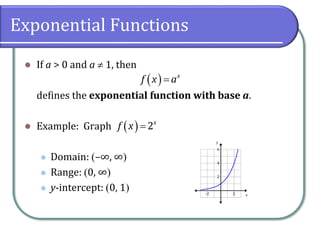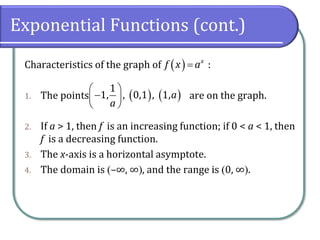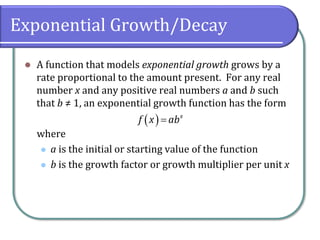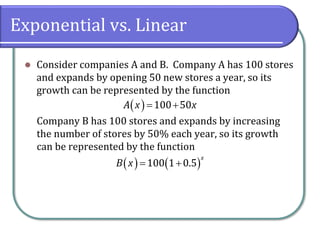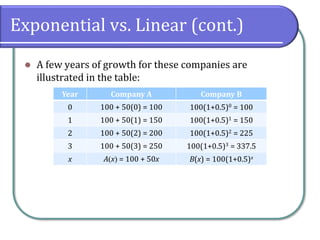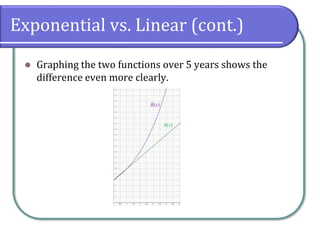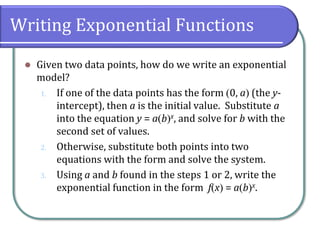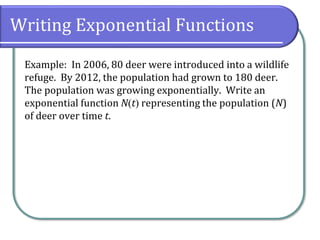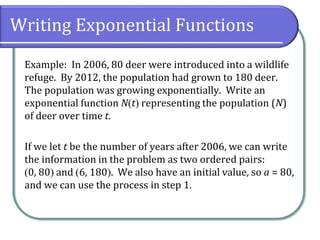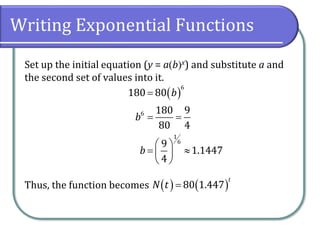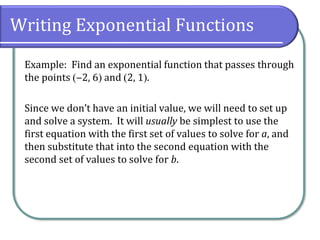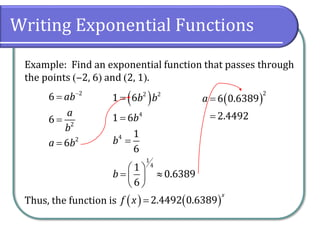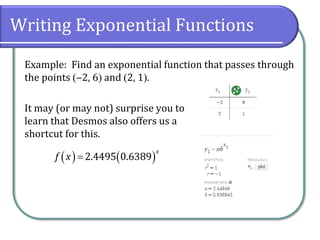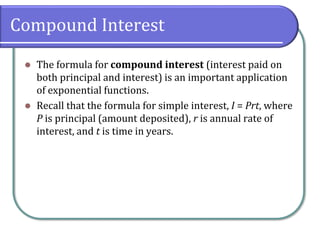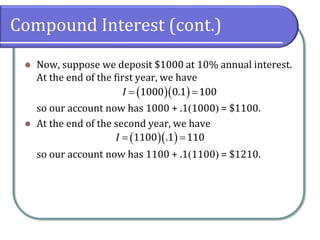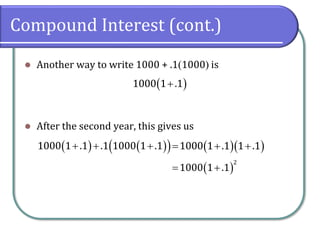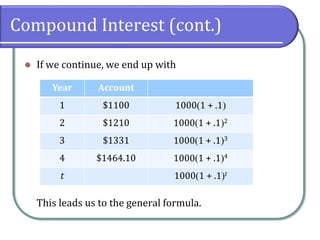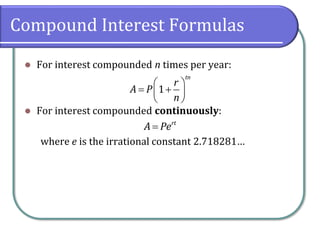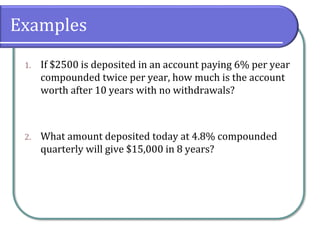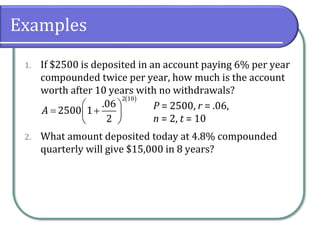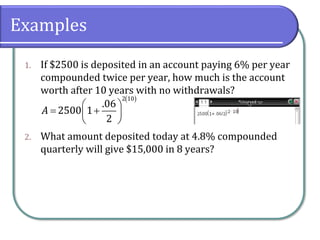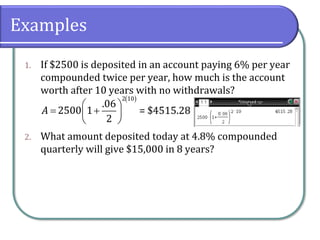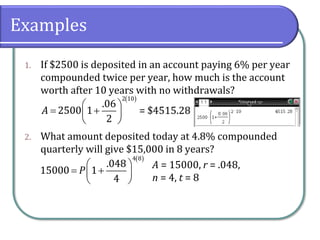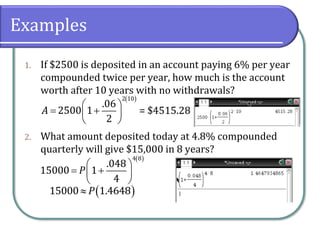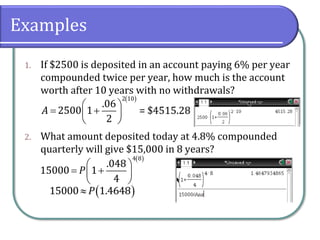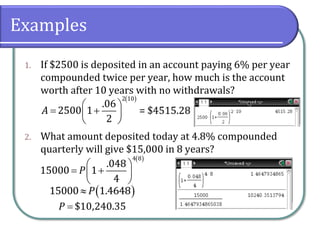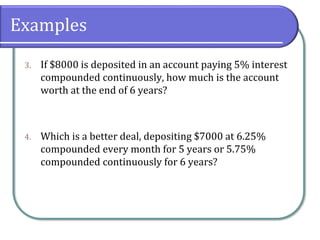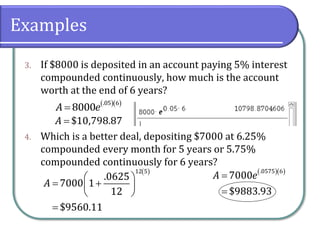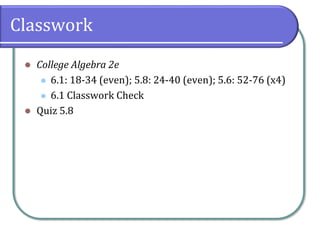1 de 34

### 6.1 Exponential Functions

• 1. Exponential Functions Chapter 6 Exponential and Logarithmic Functions
• 2. Concepts & Objectives ⚫ Exponential Functions ⚫ Evaluate exponential functions. ⚫ Find the equation of an exponential function. ⚫ Use compound interest formulas. ⚫ Evaluate exponential functions with base e.
• 3. Properties of Exponents ⚫ Recall that for a variable x and integers a and b: +  = a b a b x x x − = a a b b x x x ( ) = b a ab x x = b a b a x x =  = a b x x a b
• 4. Simplifying Exponents ⚫ Example: Simplify ⚫ 1. ⚫ 2. ⚫ 3. − 3 2 2 2 5 25 5 x y z xy z ( ) − 4 2 3 2r s t −  2 3 1 2 5 6 y y
• 5. Simplifying Exponents ⚫ Example: Simplify ⚫ 1. ⚫ 2. ⚫ 3. − 3 2 2 2 5 25 5 x y z xy z ( ) − 4 2 3 2r s t −  2 3 1 2 5 6 y y − − − − = 3 1 2 2 2 5 5x y z ( ) ( ) − = 4 2 4 3 4 4 2 r s t ( )( ) − = 2 1 3 2 5 6 y − − = 2 4 3 5x y z − = 8 12 4 16r s t = 1 6 30y
• 6. Exponential Functions ⚫ If a > 0 and a  1, then defines the exponential function with base a. ⚫ Example: Graph ⚫ Domain: (–∞, ∞) ⚫ Range: (0, ∞) ⚫ y-intercept: (0, 1) ( )= x f x a ( )=2x f x
• 7. Exponential Functions (cont.) Characteristics of the graph of : 1. The points are on the graph. 2. If a > 1, then f is an increasing function; if 0 < a < 1, then f is a decreasing function. 3. The x-axis is a horizontal asymptote. 4. The domain is (–∞, ∞), and the range is (0, ∞). ( )= x f x a ( ) ( )   −     1 1, , 0,1 , 1,a a
• 8. Exponential Growth/Decay ⚫ A function that models exponential growth grows by a rate proportional to the amount present. For any real number x and any positive real numbers a and b such that b ≠ 1, an exponential growth function has the form where ⚫ a is the initial or starting value of the function ⚫ b is the growth factor or growth multiplier per unit x ( ) x f x ab =
• 9. Exponential vs. Linear ⚫ Consider companies A and B. Company A has 100 stores and expands by opening 50 new stores a year, so its growth can be represented by the function Company B has 100 stores and expands by increasing the number of stores by 50% each year, so its growth can be represented by the function ( ) 100 50 A x x = + ( ) ( ) 100 1 0.5 x B x = +
• 10. Exponential vs. Linear (cont.) ⚫ A few years of growth for these companies are illustrated in the table: Year Company A Company B 0 100 + 50(0) = 100 100(1+0.5)0 = 100 1 100 + 50(1) = 150 100(1+0.5)1 = 150 2 100 + 50(2) = 200 100(1+0.5)2 = 225 3 100 + 50(3) = 250 100(1+0.5)3 = 337.5 x A(x) = 100 + 50x B(x) = 100(1+0.5)x
• 11. Exponential vs. Linear (cont.) ⚫ Graphing the two functions over 5 years shows the difference even more clearly. B(x) A(x)
• 12. Writing Exponential Functions ⚫ Given two data points, how do we write an exponential model? 1. If one of the data points has the form (0, a) (the y- intercept), then a is the initial value. Substitute a into the equation y = a(b)x, and solve for b with the second set of values. 2. Otherwise, substitute both points into two equations with the form and solve the system. 3. Using a and b found in the steps 1 or 2, write the exponential function in the form f(x) = a(b)x.
• 13. Writing Exponential Functions Example: In 2006, 80 deer were introduced into a wildlife refuge. By 2012, the population had grown to 180 deer. The population was growing exponentially. Write an exponential function N(t) representing the population (N) of deer over time t.
• 14. Writing Exponential Functions Example: In 2006, 80 deer were introduced into a wildlife refuge. By 2012, the population had grown to 180 deer. The population was growing exponentially. Write an exponential function N(t) representing the population (N) of deer over time t. If we let t be the number of years after 2006, we can write the information in the problem as two ordered pairs: (0, 80) and (6, 180). We also have an initial value, so a = 80, and we can use the process in step 1.
• 15. Writing Exponential Functions Set up the initial equation (y = a(b)x) and substitute a and the second set of values into it. Thus, the function becomes ( ) 6 6 1 6 180 80 180 9 80 4 9 1.1447 4 b b b = = =   =      ( ) ( ) 80 1.447 t N t =
• 16. Writing Exponential Functions Example: Find an exponential function that passes through the points (‒2, 6) and (2, 1). Since we don’t have an initial value, we will need to set up and solve a system. It will usually be simplest to use the first equation with the first set of values to solve for a, and then substitute that into the second equation with the second set of values to solve for b.
• 17. Writing Exponential Functions Example: Find an exponential function that passes through the points (‒2, 6) and (2, 1). Thus, the function is 2 2 2 6 6 6 ab a b a b − = = = ( ) 2 2 4 4 1 4 1 6 1 6 1 6 1 0.6389 6 b b b b b = = =   =      ( ) 2 6 0.6389 2.4492 a = = ( ) ( ) 2.4492 0.6389 x f x =
• 18. Writing Exponential Functions Example: Find an exponential function that passes through the points (‒2, 6) and (2, 1). It may (or may not) surprise you to learn that Desmos also offers us a shortcut for this. ( ) ( ) 2.4495 0.6389 x f x =
• 19. Compound Interest ⚫ The formula for compound interest (interest paid on both principal and interest) is an important application of exponential functions. ⚫ Recall that the formula for simple interest, I = Prt, where P is principal (amount deposited), r is annual rate of interest, and t is time in years.
• 20. Compound Interest (cont.) ⚫ Now, suppose we deposit \$1000 at 10% annual interest. At the end of the first year, we have so our account now has 1000 + .1(1000) = \$1100. ⚫ At the end of the second year, we have so our account now has 1100 + .1(1100) = \$1210. ( )( ) = = 1000 0.1 100 I ( )( ) = = 1100 .1 110 I
• 21. Compound Interest (cont.) ⚫ Another way to write 1000 + .1(1000) is ⚫ After the second year, this gives us ( ) ( ) ( ) + + + 1000 1 .1 .1 1000 1 .1 ( )( ) = + + 1000 1 .1 1 .1 ( ) = + 2 1000 1 .1 ( ) + 1000 1 .1
• 22. Compound Interest (cont.) ⚫ If we continue, we end up with This leads us to the general formula. Year Account 1 \$1100 1000(1 + .1) 2 \$1210 1000(1 + .1)2 3 \$1331 1000(1 + .1)3 4 \$1464.10 1000(1 + .1)4 t 1000(1 + .1)t
• 23. Compound Interest Formulas ⚫ For interest compounded n times per year: ⚫ For interest compounded continuously: where e is the irrational constant 2.718281…   = +     1 tn r A P n = rt A Pe
• 24. Examples 1. If \$2500 is deposited in an account paying 6% per year compounded twice per year, how much is the account worth after 10 years with no withdrawals? 2. What amount deposited today at 4.8% compounded quarterly will give \$15,000 in 8 years?
• 25. Examples 1. If \$2500 is deposited in an account paying 6% per year compounded twice per year, how much is the account worth after 10 years with no withdrawals? 2. What amount deposited today at 4.8% compounded quarterly will give \$15,000 in 8 years? ( )   = +     2 10 .06 2500 1 2 A P = 2500, r = .06, n = 2, t = 10
• 26. Examples 1. If \$2500 is deposited in an account paying 6% per year compounded twice per year, how much is the account worth after 10 years with no withdrawals? 2. What amount deposited today at 4.8% compounded quarterly will give \$15,000 in 8 years? ( )   = +     2 10 .06 2500 1 2 A
• 27. Examples 1. If \$2500 is deposited in an account paying 6% per year compounded twice per year, how much is the account worth after 10 years with no withdrawals? 2. What amount deposited today at 4.8% compounded quarterly will give \$15,000 in 8 years? ( )   = +     2 10 .06 2500 1 2 A = \$4515.28
• 28. Examples 1. If \$2500 is deposited in an account paying 6% per year compounded twice per year, how much is the account worth after 10 years with no withdrawals? 2. What amount deposited today at 4.8% compounded quarterly will give \$15,000 in 8 years? ( )   = +     2 10 .06 2500 1 2 A ( )   = +     4 8 .048 15000 1 4 P A = 15000, r = .048, n = 4, t = 8 = \$4515.28
• 29. Examples 1. If \$2500 is deposited in an account paying 6% per year compounded twice per year, how much is the account worth after 10 years with no withdrawals? 2. What amount deposited today at 4.8% compounded quarterly will give \$15,000 in 8 years? ( )   = +     2 10 .06 2500 1 2 A ( )   = +     4 8 .048 15000 1 4 P ( )  15000 1.4648 P = \$4515.28
• 30. Examples 1. If \$2500 is deposited in an account paying 6% per year compounded twice per year, how much is the account worth after 10 years with no withdrawals? 2. What amount deposited today at 4.8% compounded quarterly will give \$15,000 in 8 years? ( )   = +     2 10 .06 2500 1 2 A ( )   = +     4 8 .048 15000 1 4 P ( )  15000 1.4648 P = \$4515.28
• 31. Examples 1. If \$2500 is deposited in an account paying 6% per year compounded twice per year, how much is the account worth after 10 years with no withdrawals? 2. What amount deposited today at 4.8% compounded quarterly will give \$15,000 in 8 years? ( )   = +     2 10 .06 2500 1 2 A ( )   = +     4 8 .048 15000 1 4 P ( )  15000 1.4648 P = \$10,240.35 P = \$4515.28
• 32. Examples 3. If \$8000 is deposited in an account paying 5% interest compounded continuously, how much is the account worth at the end of 6 years? 4. Which is a better deal, depositing \$7000 at 6.25% compounded every month for 5 years or 5.75% compounded continuously for 6 years?
• 33. Examples 3. If \$8000 is deposited in an account paying 5% interest compounded continuously, how much is the account worth at the end of 6 years? 4. Which is a better deal, depositing \$7000 at 6.25% compounded every month for 5 years or 5.75% compounded continuously for 6 years? ( )( ) = .05 6 8000 A e = \$10,798.87 A ( )   = +     = 12 5 .0625 7000 1 12 \$9560.11 A ( )( ) = = .0575 6 7000 \$9883.93 A e
• 34. Classwork ⚫ College Algebra 2e ⚫ 6.1: 18-34 (even); 5.8: 24-40 (even); 5.6: 52-76 (x4) ⚫ 6.1 Classwork Check ⚫ Quiz 5.8